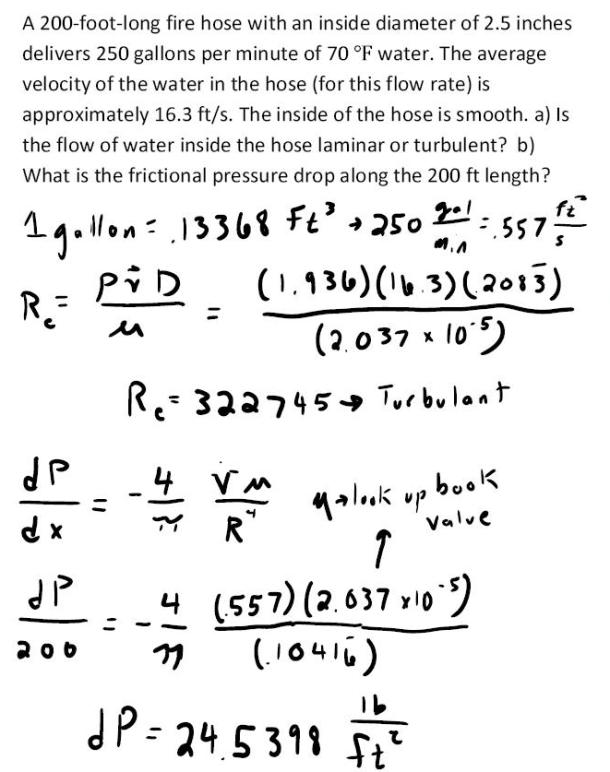fluid mechanics math problems engineering equations fluid mechanics basic mechanics school homework engineering math fluids formulas fluid mechanics problems fluid mechanics problem solutions to fluids problems full solution fluid mechanics engineering fluids problem solution fluid mechanics math problems engineering equations fluid mechanics basic mechanics school homework engineering math fluid mechanics formulas fluid problems fluid mechanics problem solutions to fluid mechanics problems full solution fluid mechanics engineering fluid mechanics problem solution
fluid mechanics math problems engineering equations fluid mechanics basic mechanics school homework engineering math fluids formulas fluid mechanics problems fluid mechanics problem solutions to fluids problems full solution fluid mechanics engineering fluids problem solution fluid mechanics math problems engineering equations fluid mechanics basic mechanics school homework engineering math fluid mechanics formulas fluid problems fluid mechanics problem solutions to fluid mechanics problems full solution fluid mechanics engineering fluid mechanics problem solution
Highalphabet Home Page fluid mechanics solutions fluids math solved Fluid Mechanics Page 1
A 200 foot long fire hose with an inside diameter of 2.5 inches delivers 250 gallons per minute of 70 degree F water. The average velocity of the water in the hose (for this flow rate) is approximately 16.3 ft/s. The inside of the hose is smooth. a) Is the flow of water inside the hose laminar or turbulent? b) What is the frictional pressure drop along the 200 ft length?A 200 foot long fire hose with an inside diameter of 2.5 inches delivers 250 gallons per minute of 70 degree F water. The average velocity of the water in the hose (for this flow rate) is approximately 16.3 ft/s. The inside of the hose is smooth. a) Is the flow of water inside the hose laminar or turbulent? b) What is the frictional pressure drop along the 200 ft length?